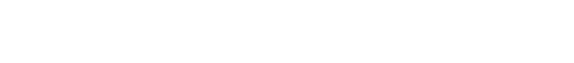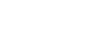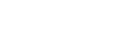## 凸集（Convex Sets）## Epigraph

epigraph 是在函数中或其上的一组点。## 凸函数（Convex Function）## 凸性检验```import numpy as np
import matplotlib.pyplot as plt
x=np.linspace(-1, 2, 100)
# Convex function y1
y1 = np.exp(x)
plt.figure(1)
plt.plot(x, y1)
plt.xlabel('\$x\$')
plt.ylabel('\$e^x\$')
plt.show()
# Concave function y2
y2 = -np.exp(x)
plt.figure(2)
plt.plot(x, y2)
plt.xlabel('\$x\$')
plt.ylabel('\$-e^x\$')
plt.show()
view raw```## 梯度下降法优化中的凸性MSE 方程